# ISEE Upper Level Math : How to find the angle for a percentage of a circle

## Example Questions

### Example Question #1 : Sectors

Sector TYP occupies 43% of a circle. Find the degree measure of angle TYP.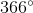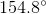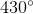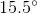Explanation:

Sector TYP occupies 43% of a circle. Find the degree measure of angle TYP.

Use the following formula and solve for x: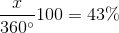Begin by dividing over the 100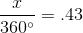Then multiply by 360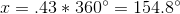### Example Question #2 : Sectors

If sector AJL covers 45% of circle J, what is the measure of sector AJL's central angle?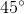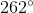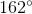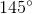Explanation:

If sector AJL covers 45% of circle J, what is the measure of sector AJL's central angle?

To find an angle measure from a percentage, simply convert the percentage to a decimal and then multiply it by 360 degrees.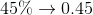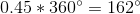So, our answer is 162 degrees.